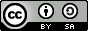# NandakumarContent Network

## Simple Interest Calculator Using JavaScript

By | |Let us create a simple web app that helps to calculate simple interest. The code is given below. You can paste it into a text editor (e.g.: gEdit or Notepad), save it with a filename that has the extension .html (e.g.: simpleint.html), open it in a web browser, and run it.

```<html> <head> <title>Web App for Simple Interest Calculation</title> <script> function calculate() { p = document.getElementById("p").value; n = document.getElementById("n").value; r = document.getElementById("r").value; result = document.getElementById("result"); result.innerHTML = "The interest is " + (p*n*r/100); } </script> </head> <body> <h1>Simple Interest Calculation</h1> Amount: <input id="p"><br/> Rate: <input id="r"><br/> No. of Years: <input id="n"><br/> <button onclick="calculate()">Calculate</button> <p id="result"></p> </body> </html>```

The `calculate()` function acts as the handler for the `onclick` event of the button. That is, when the user clicks the button Calculate, the `calculate()` function is executed.

The function should get the values that the user has entered into the input boxes. In order to do this, it uses the `document.getElementById()` method and the `value` property. After the calculation, the result is put inside a paragraph element using the same mechanism (assignment instead of reading, this time).

Here it works:

##### Simple Interest Calculation
Amount:
Rate:
No. of Years:

Simple Interest Calculator Using JavaScript | Nandakumar Content Network